# Mean State

Period Mean (original grids) [W/m2]
Model Period Mean (intersection) [W/m2]
Model Period Mean (complement) [W/m2]
Benchmark Period Mean (intersection) [W/m2]
Benchmark Period Mean (complement) [W/m2]
Bias [W/m2]
RMSE [W/m2]
Phase Shift [months]
Bias Score 
RMSE Score 
Seasonal Cycle Score 
Spatial Distribution Score 
Interannual Variability Score 
Overall Score 
Benchmark [-] 187.
CRUNCEPv7 [-] 192. 192. 0.00 186. 206. 5.24 16.6 1.20 0.63 0.48 0.84 0.97 0.48 0.65
GSWP3v1 [-] 182. 182. 0.00 186. 206. -4.45 15.1 0.823 0.73 0.49 0.90 0.94 0.80 0.73
WATCH [-] 141. 141. 0.00 185. 208. -42.4 48.5 1.82 0.17 0.39 0.73 0.87 0.58 0.52
Period Mean (original grids) [W/m2]
Model Period Mean (intersection) [W/m2]
Model Period Mean (complement) [W/m2]
Benchmark Period Mean (intersection) [W/m2]
Benchmark Period Mean (complement) [W/m2]
Bias [W/m2]
RMSE [W/m2]
Phase Shift [months]
Bias Score 
RMSE Score 
Seasonal Cycle Score 
Spatial Distribution Score 
Interannual Variability Score 
Overall Score 
Benchmark [-] 198.
CRUNCEPv7 [-] 198. 198. 0.00 198. 214. 0.401 18.8 1.49 0.57 0.47 0.78 0.95 0.74 0.66
GSWP3v1 [-] 195. 195. 0.00 198. 214. -2.80 14.2 0.767 0.71 0.52 0.91 0.96 0.85 0.74
WATCH [-] 160. 161. 0.00 198. 213. -36.0 40.6 1.02 0.23 0.48 0.88 0.90 0.59 0.59
Period Mean (original grids) [W/m2]
Model Period Mean (intersection) [W/m2]
Model Period Mean (complement) [W/m2]
Benchmark Period Mean (intersection) [W/m2]
Benchmark Period Mean (complement) [W/m2]
Bias [W/m2]
RMSE [W/m2]
Phase Shift [months]
Bias Score 
RMSE Score 
Seasonal Cycle Score 
Spatial Distribution Score 
Interannual Variability Score 
Overall Score 
Benchmark [-] 199.
CRUNCEPv7 [-] 195. 195. 0.00 198. 219. -3.72 17.4 1.18 0.71 0.55 0.83 0.87 0.53 0.67
GSWP3v1 [-] 196. 196. 0.00 198. 219. -2.87 15.6 0.787 0.80 0.57 0.89 1.0 0.75 0.76
WATCH [-] 179. 179. 0.00 195. 213. -16.1 25.4 1.42 0.52 0.52 0.80 0.94 0.64 0.66
Period Mean (original grids) [W/m2]
Model Period Mean (intersection) [W/m2]
Model Period Mean (complement) [W/m2]
Benchmark Period Mean (intersection) [W/m2]
Benchmark Period Mean (complement) [W/m2]
Bias [W/m2]
RMSE [W/m2]
Phase Shift [months]
Bias Score 
RMSE Score 
Seasonal Cycle Score 
Spatial Distribution Score 
Interannual Variability Score 
Overall Score 
Benchmark [-] 70.7
CRUNCEPv7 [-] 75.8 75.9 0.00 70.5 72.8 3.73 21.2 0.340 0.87 0.76 0.98 0.97 0.68 0.83
GSWP3v1 [-] 73.1 73.3 0.00 70.5 72.8 1.86 18.4 0.331 0.91 0.77 0.98 1.0 0.74 0.86
WATCH [-] 65.6 65.7 0.00 74.7 74.7 -9.24 17.8 0.477 0.86 0.77 0.97 0.99 0.69 0.84
Period Mean (original grids) [W/m2]
Model Period Mean (intersection) [W/m2]
Model Period Mean (complement) [W/m2]
Benchmark Period Mean (intersection) [W/m2]
Benchmark Period Mean (complement) [W/m2]
Bias [W/m2]
RMSE [W/m2]
Phase Shift [months]
Bias Score 
RMSE Score 
Seasonal Cycle Score 
Spatial Distribution Score 
Interannual Variability Score 
Overall Score 
Benchmark [-] 194.
CRUNCEPv7 [-] 186. 186. 0.00 190. 207. -1.72 18.0 1.47 0.61 0.44 0.78 0.84 0.52 0.61
GSWP3v1 [-] 175. 175. 0.00 190. 207. -11.2 19.1 1.25 0.54 0.44 0.80 0.96 0.77 0.66
WATCH [-] 138. 137. 0.00 189. 203. -43.7 48.5 2.01 0.16 0.33 0.68 0.56 0.57 0.44
Period Mean (original grids) [W/m2]
Model Period Mean (intersection) [W/m2]
Model Period Mean (complement) [W/m2]
Benchmark Period Mean (intersection) [W/m2]
Benchmark Period Mean (complement) [W/m2]
Bias [W/m2]
RMSE [W/m2]
Phase Shift [months]
Bias Score 
RMSE Score 
Seasonal Cycle Score 
Spatial Distribution Score 
Interannual Variability Score 
Overall Score 
Benchmark [-] 97.8
CRUNCEPv7 [-] 108. 108. 0.00 97.6 118. 11.0 20.9 0.266 0.85 0.79 0.98 1.0 0.72 0.86
GSWP3v1 [-] 100. 100. 0.00 97.6 118. 2.99 15.5 0.231 0.92 0.82 0.98 1.0 0.69 0.87
WATCH [-] 87.3 87.4 0.00 102. 119. -14.4 16.1 0.320 0.81 0.81 0.98 0.99 0.73 0.86
Period Mean (original grids) [W/m2]
Model Period Mean (intersection) [W/m2]
Model Period Mean (complement) [W/m2]
Benchmark Period Mean (intersection) [W/m2]
Benchmark Period Mean (complement) [W/m2]
Bias [W/m2]
RMSE [W/m2]
Phase Shift [months]
Bias Score 
RMSE Score 
Seasonal Cycle Score 
Spatial Distribution Score 
Interannual Variability Score 
Overall Score 
Benchmark [-] 139.
CRUNCEPv7 [-] 149. 149. 0.00 138. 149. 10.6 23.3 0.448 0.76 0.75 0.96 0.97 0.72 0.82
GSWP3v1 [-] 135. 135. 0.00 138. 149. -2.61 15.9 0.340 0.88 0.77 0.97 1.0 0.67 0.84
WATCH [-] 131. 131. 0.00 143. 155. -11.8 20.3 0.636 0.79 0.72 0.93 0.98 0.71 0.81
Period Mean (original grids) [W/m2]
Model Period Mean (intersection) [W/m2]
Model Period Mean (complement) [W/m2]
Benchmark Period Mean (intersection) [W/m2]
Benchmark Period Mean (complement) [W/m2]
Bias [W/m2]
RMSE [W/m2]
Phase Shift [months]
Bias Score 
RMSE Score 
Seasonal Cycle Score 
Spatial Distribution Score 
Interannual Variability Score 
Overall Score 
Benchmark [-] 181.
CRUNCEPv7 [-] 193. 193. 0.00 179. 205. 13.7 25.4 0.335 0.58 0.59 0.97 0.96 0.65 0.72
GSWP3v1 [-] 174. 174. 0.00 179. 205. -3.72 18.1 0.320 0.77 0.61 0.97 0.94 0.72 0.77
WATCH [-] 159. 159. 0.00 180. 203. -19.6 30.1 0.645 0.54 0.52 0.93 0.93 0.72 0.69
Period Mean (original grids) [W/m2]
Model Period Mean (intersection) [W/m2]
Model Period Mean (complement) [W/m2]
Benchmark Period Mean (intersection) [W/m2]
Benchmark Period Mean (complement) [W/m2]
Bias [W/m2]
RMSE [W/m2]
Phase Shift [months]
Bias Score 
RMSE Score 
Seasonal Cycle Score 
Spatial Distribution Score 
Interannual Variability Score 
Overall Score 
Benchmark [-] 62.8
CRUNCEPv7 [-] 68.8 69.2 0.00 63.2 54.4 4.51 19.9 0.302 0.89 0.76 0.98 0.94 0.72 0.84
GSWP3v1 [-] 64.7 65.0 0.00 63.2 54.4 0.684 15.6 0.199 0.93 0.79 0.99 0.99 0.75 0.87
WATCH [-] 53.2 53.5 0.00 68.8 57.9 -16.0 19.4 0.173 0.79 0.79 0.99 0.96 0.69 0.83
Period Mean (original grids) [W/m2]
Model Period Mean (intersection) [W/m2]
Model Period Mean (complement) [W/m2]
Benchmark Period Mean (intersection) [W/m2]
Benchmark Period Mean (complement) [W/m2]
Bias [W/m2]
RMSE [W/m2]
Phase Shift [months]
Bias Score 
RMSE Score 
Seasonal Cycle Score 
Spatial Distribution Score 
Interannual Variability Score 
Overall Score 
Benchmark [-] 198.
CRUNCEPv7 [-] 196. 196. 0.00 198. 197. -4.02 14.4 0.511 0.85 0.78 0.97 0.98 0.66 0.83
GSWP3v1 [-] 192. 192. 0.00 198. 197. -7.59 15.6 0.385 0.85 0.76 0.97 1.0 0.72 0.84
WATCH [-] 195. 195. 0.00 193. 189. 0.443 14.4 0.589 0.86 0.75 0.94 1.0 0.79 0.85
Period Mean (original grids) [W/m2]
Model Period Mean (intersection) [W/m2]
Model Period Mean (complement) [W/m2]
Benchmark Period Mean (intersection) [W/m2]
Benchmark Period Mean (complement) [W/m2]
Bias [W/m2]
RMSE [W/m2]
Phase Shift [months]
Bias Score 
RMSE Score 
Seasonal Cycle Score 
Spatial Distribution Score 
Interannual Variability Score 
Overall Score 
Benchmark [-] 120.
CRUNCEPv7 [-] 132. 132. 0.00 118. 145. 14.6 24.3 0.228 0.77 0.80 0.98 0.99 0.73 0.85
GSWP3v1 [-] 113. 113. 0.00 118. 145. -2.30 13.6 0.227 0.92 0.84 0.98 0.99 0.66 0.87
WATCH [-] 95.7 95.7 0.00 121. 150. -22.2 21.7 0.452 0.73 0.82 0.97 0.95 0.71 0.83
Period Mean (original grids) [W/m2]
Model Period Mean (intersection) [W/m2]
Model Period Mean (complement) [W/m2]
Benchmark Period Mean (intersection) [W/m2]
Benchmark Period Mean (complement) [W/m2]
Bias [W/m2]
RMSE [W/m2]
Phase Shift [months]
Bias Score 
RMSE Score 
Seasonal Cycle Score 
Spatial Distribution Score 
Interannual Variability Score 
Overall Score 
Benchmark [-] 163.
CRUNCEPv7 [-] 144. 144. 0.00 142. 173. 1.68 19.7 0.586 0.75 0.69 0.93 0.99 0.61 0.78
GSWP3v1 [-] 137. 137. 0.00 142. 173. -4.59 16.0 0.394 0.82 0.70 0.96 1.0 0.71 0.82
WATCH [-] 126. 126. 0.00 143. 172. -16.8 23.9 0.697 0.65 0.67 0.91 0.99 0.66 0.76
Period Mean (original grids) [W/m2]
Model Period Mean (intersection) [W/m2]
Model Period Mean (complement) [W/m2]
Benchmark Period Mean (intersection) [W/m2]
Benchmark Period Mean (complement) [W/m2]
Bias [W/m2]
RMSE [W/m2]
Phase Shift [months]
Bias Score 
RMSE Score 
Seasonal Cycle Score 
Spatial Distribution Score 
Interannual Variability Score 
Overall Score 
Benchmark [-] 177.
CRUNCEPv7 [-] 168. 168. 0.00 175. 217. -6.31 17.8 0.410 0.72 0.75 0.96 0.98 0.71 0.81
GSWP3v1 [-] 162. 162. 0.00 175. 217. -12.0 18.0 0.296 0.71 0.76 0.98 0.96 0.67 0.81
WATCH [-] 147. 147. 0.00 180. 222. -31.6 30.2 0.411 0.45 0.77 0.96 0.99 0.62 0.76
Period Mean (original grids) [W/m2]
Model Period Mean (intersection) [W/m2]
Model Period Mean (complement) [W/m2]
Benchmark Period Mean (intersection) [W/m2]
Benchmark Period Mean (complement) [W/m2]
Bias [W/m2]
RMSE [W/m2]
Phase Shift [months]
Bias Score 
RMSE Score 
Seasonal Cycle Score 
Spatial Distribution Score 
Interannual Variability Score 
Overall Score 
Benchmark [-] 69.7
CRUNCEPv7 [-] 75.4 75.2 0.00 69.6 71.1 3.21 20.9 0.131 0.88 0.75 0.99 0.91 0.61 0.82
GSWP3v1 [-] 70.7 70.6 0.00 69.6 71.1 -0.616 17.1 0.0938 0.92 0.78 0.99 0.98 0.75 0.87
WATCH [-] 66.1 65.9 0.00 73.0 74.1 -8.19 18.5 0.207 0.86 0.77 0.99 0.99 0.69 0.84
Period Mean (original grids) [W/m2]
Model Period Mean (intersection) [W/m2]
Model Period Mean (complement) [W/m2]
Benchmark Period Mean (intersection) [W/m2]
Benchmark Period Mean (complement) [W/m2]
Bias [W/m2]
RMSE [W/m2]
Phase Shift [months]
Bias Score 
RMSE Score 
Seasonal Cycle Score 
Spatial Distribution Score 
Interannual Variability Score 
Overall Score 
Benchmark [-] 204.
CRUNCEPv7 [-] 190. 190. 0.00 201. 219. -9.17 24.0 0.944 0.61 0.64 0.91 0.79 0.67 0.71
GSWP3v1 [-] 192. 192. 0.00 201. 219. -6.91 16.8 0.470 0.77 0.68 0.94 0.99 0.75 0.80
WATCH [-] 181. 181. 0.00 202. 221. -20.1 29.1 0.893 0.55 0.64 0.87 0.85 0.77 0.72
Period Mean (original grids) [W/m2]
Model Period Mean (intersection) [W/m2]
Model Period Mean (complement) [W/m2]
Benchmark Period Mean (intersection) [W/m2]
Benchmark Period Mean (complement) [W/m2]
Bias [W/m2]
RMSE [W/m2]
Phase Shift [months]
Bias Score 
RMSE Score 
Seasonal Cycle Score 
Spatial Distribution Score 
Interannual Variability Score 
Overall Score 
Benchmark [-] 150.
CRUNCEPv7 [-] 155. 155. 0.00 148. 192. 7.59 17.9 0.415 0.84 0.78 0.97 0.98 0.58 0.82
GSWP3v1 [-] 145. 145. 0.00 148. 192. -2.06 13.6 0.274 0.94 0.80 0.98 0.99 0.70 0.87
WATCH [-] 147. 147. 0.00 151. 197. -3.24 15.9 0.399 0.88 0.77 0.97 0.98 0.68 0.84
Period Mean (original grids) [W/m2]
Model Period Mean (intersection) [W/m2]
Model Period Mean (complement) [W/m2]
Benchmark Period Mean (intersection) [W/m2]
Benchmark Period Mean (complement) [W/m2]
Bias [W/m2]
RMSE [W/m2]
Phase Shift [months]
Bias Score 
RMSE Score 
Seasonal Cycle Score 
Spatial Distribution Score 
Interannual Variability Score 
Overall Score 
Benchmark [-] 110.
CRUNCEPv7 [-] 122. 123. 0.00 110. 117. 12.7 21.6 0.262 0.83 0.80 0.98 0.97 0.56 0.82
GSWP3v1 [-] 111. 111. 0.00 110. 117. 1.63 13.7 0.114 0.93 0.84 0.99 1.0 0.68 0.88
WATCH [-] 109. 109. 0.00 114. 124. -5.43 13.5 0.374 0.90 0.82 0.97 0.99 0.73 0.87
Period Mean (original grids) [W/m2]
Model Period Mean (intersection) [W/m2]
Model Period Mean (complement) [W/m2]
Benchmark Period Mean (intersection) [W/m2]
Benchmark Period Mean (complement) [W/m2]
Bias [W/m2]
RMSE [W/m2]
Phase Shift [months]
Bias Score 
RMSE Score 
Seasonal Cycle Score 
Spatial Distribution Score 
Interannual Variability Score 
Overall Score 
Benchmark [-] 161.
CRUNCEPv7 [-] 154. 154. 0.00 161. 163. -6.92 17.1 0.227 0.88 0.81 0.98 0.95 0.61 0.84
GSWP3v1 [-] 159. 159. 0.00 161. 163. -1.43 14.3 0.202 0.93 0.82 0.99 0.98 0.70 0.87
WATCH [-] 163. 163. 0.00 167. 167. -3.13 16.2 0.273 0.90 0.80 0.98 0.98 0.71 0.86
Period Mean (original grids) [W/m2]
Model Period Mean (intersection) [W/m2]
Model Period Mean (complement) [W/m2]
Benchmark Period Mean (intersection) [W/m2]
Benchmark Period Mean (complement) [W/m2]
Bias [W/m2]
RMSE [W/m2]
Phase Shift [months]
Bias Score 
RMSE Score 
Seasonal Cycle Score 
Spatial Distribution Score 
Interannual Variability Score 
Overall Score 
Benchmark [-] 179.
CRUNCEPv7 [-] 182. 182. 0.00 178. 218. 5.20 17.0 1.48 0.61 0.45 0.78 0.82 0.58 0.61
GSWP3v1 [-] 173. 173. 0.00 178. 218. -3.71 14.5 0.798 0.73 0.45 0.90 0.96 0.80 0.71
WATCH [-] 152. 152. 0.00 178. 219. -25.8 32.8 1.74 0.27 0.37 0.75 0.95 0.55 0.54
Period Mean (original grids) [W/m2]
Model Period Mean (intersection) [W/m2]
Model Period Mean (complement) [W/m2]
Benchmark Period Mean (intersection) [W/m2]
Benchmark Period Mean (complement) [W/m2]
Bias [W/m2]
RMSE [W/m2]
Phase Shift [months]
Bias Score 
RMSE Score 
Seasonal Cycle Score 
Spatial Distribution Score 
Interannual Variability Score 
Overall Score 
Benchmark [-] 185.
CRUNCEPv7 [-] 186. 186. 0.00 184. 202. 1.52 20.3 0.964 0.68 0.62 0.88 0.85 0.54 0.70
GSWP3v1 [-] 180. 180. 0.00 184. 202. -4.33 15.8 0.702 0.82 0.64 0.91 0.97 0.72 0.78
WATCH [-] 162. 163. 0.00 179. 198. -16.6 25.5 1.34 0.57 0.60 0.79 0.85 0.67 0.68
Period Mean (original grids) [W/m2]
Model Period Mean (intersection) [W/m2]
Model Period Mean (complement) [W/m2]
Benchmark Period Mean (intersection) [W/m2]
Benchmark Period Mean (complement) [W/m2]
Bias [W/m2]
RMSE [W/m2]
Phase Shift [months]
Bias Score 
RMSE Score 
Seasonal Cycle Score 
Spatial Distribution Score 
Interannual Variability Score 
Overall Score 
Benchmark [-] 178.
CRUNCEPv7 [-] 178. 178. 0.00 179. 169. -4.32 27.3 0.588 0.68 0.69 0.94 0.80 0.64 0.74
GSWP3v1 [-] 176. 175. 0.00 179. 169. -8.13 21.0 0.401 0.78 0.71 0.96 1.0 0.69 0.81
WATCH [-] 173. 172. 0.00 174. 162. -5.80 26.3 0.513 0.71 0.70 0.94 0.99 0.69 0.79

# Temporally integrated period mean

BENCHMARK MEAN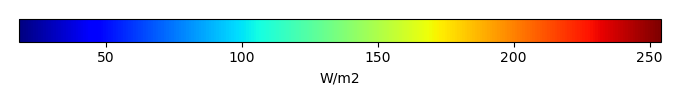MODEL MEANBIAS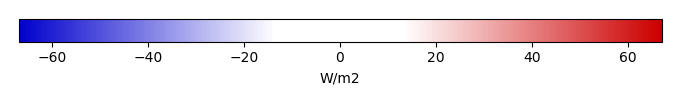BIAS SCORERMSE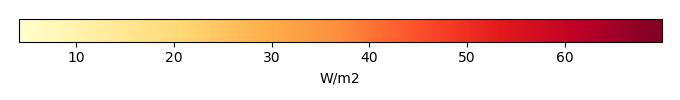RMSE SCOREBENCHMARK INTERANNUAL VARIABILITY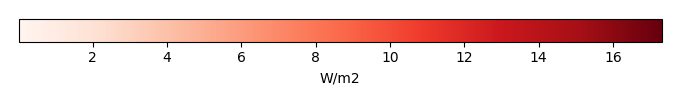MODEL INTERANNUAL VARIABILITYINTERANNUAL VARIABILITY SCOREBENCHMARK MAX MONTHMODEL MAX MONTHDIFFERENCE IN MAX MONTH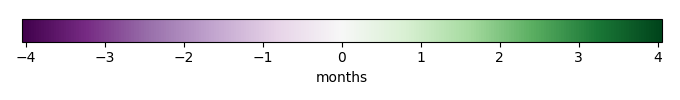SEASONAL CYCLE SCORESPATIAL TAYLOR DIAGRAMMODEL COLORS# Spatially integrated regional mean

MODEL COLORSREGIONAL MEANANNUAL CYCLEMONTHLY ANOMALYANNUAL CYCLE# All Models

BenchmarkCRUNCEPv7GSWP3v1WATCH# Data Information

creation_date: Thu May 8 23:03:44 PDT 2014

source_file: This product is generated from monthly 1 degree CERES EBAF Radiation observations